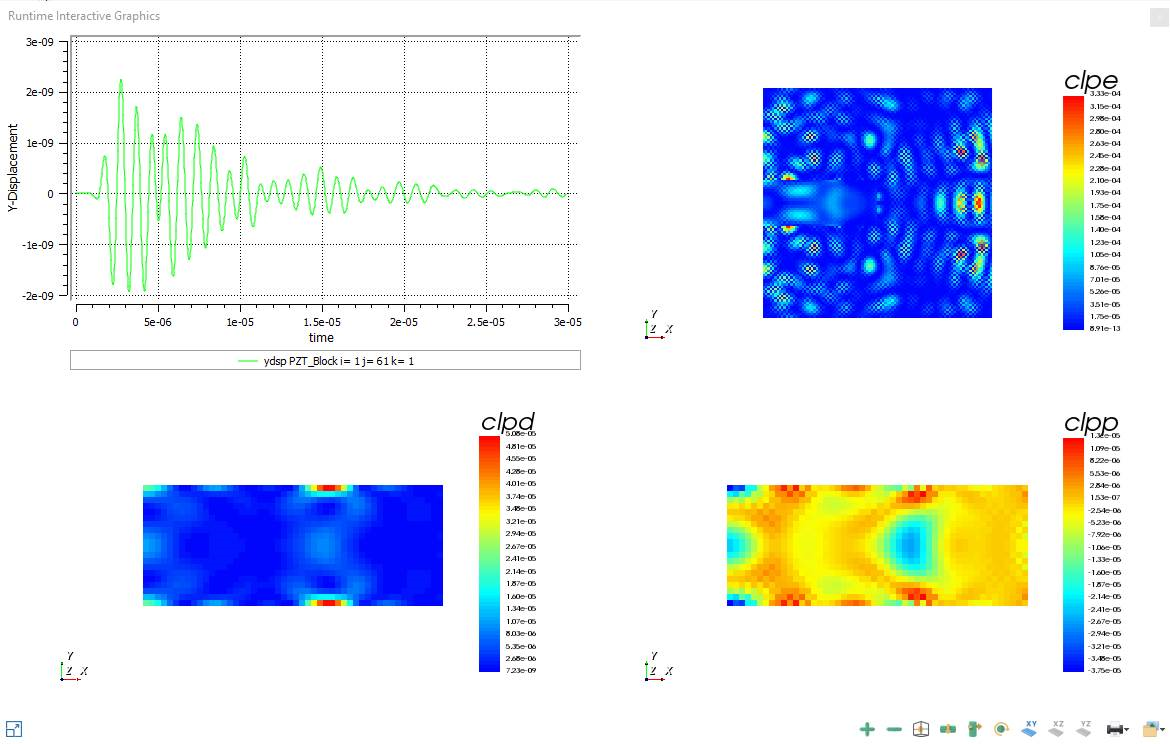# Calculating Energy Integrals with CALC PZENRG

## The 4 Types of Energy Integrals

Kinetic Energy is created by to the movement of the elemental domains in the model; Elastic Energy (or potential or strain) is the energy that is stored in the deformed structure, much like a spring. These two are the exact equivalents of a harmonic oscillator in a lumped system, and energy converts back and forth between the two.

When an electric field is applied, the material will also store Electric Energy, like a capacitor.

In case of a non-piezo material, there are these 3 energies, but there is no coupling between the electric and mechanical ones as there is no mechanism to support this.

For a piezoelectric material, however, there is coupling between the E fields and stress (virtually stiffens the material) that is an extra form of energy called Coupling Energy.

## How does OnScale calculates Energy integrals?

OnScale can calculate 'energy integrals' that is, splitting the energy into its component parts (piezoelectric, dielectric, kinetic and strain) and summing over a region (often the entire model).

This allows the user to analyse the transducer in more detail with respect to energy transmission, reflection, and efficiency in general.

Note: The boundary work surface (bwork command) is used to to calculate the energy flux or instantaneous acoustic power through a specified line/plane.

Warning: BWORK is only implemented on the model boundaries.

The command "calc pzenrg" calculate the various energies for piezoelectric models:

• Kinetic Energy
• Dielectric Energy
• Elastic Energy
• Coupling Energy

Kinetic energy per unit volume, dielectric energy, elastic energy and coupling energy are calculated as: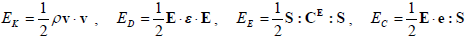Where v are the velocities, ρ is the mass density, E the electric fields, S the strains, e the coupling coefficients, ε the dielectric tensor and CE the Elastic stiffness at zero electric field.

## How to define the model for energy integral calculation with calc pzengr?

In order to calculate the Kinetic energy per unit volume, dielectric energy, elastic energy and coupling energy (Respectively arrays "clpk", "clpd", "clpe" and "clpp" ), the user needs to:

### 1- In "calc" command add "strn", "loss" and "pzenrg"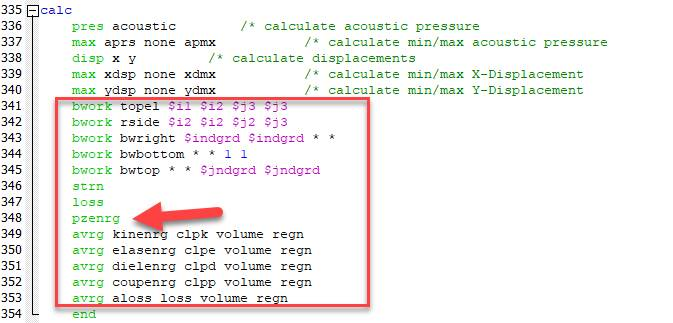Note: For all types of losses / energies added integrals over the whole domain / surface. Used the average (calc-avrg) command for this; when exporting with the 's' option, it exports the sum (integral) rather than the actual average

### 2- In "piez" command add "calc pzenrg" at the end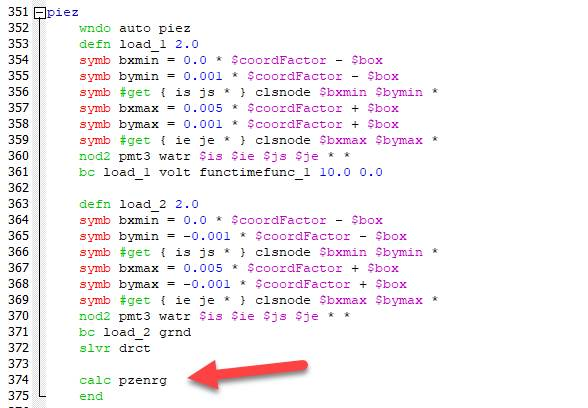### 3- To Vizualize, in the runtime grph loop, add "clpe, clpd, clpp"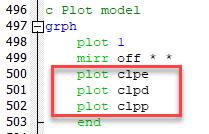Note: Dielectric energy and coupling energy are not mapped onto the ijk lattice. Because of that, you cannot visualize clpd and clpp in the flxdato file. You can only vizualize in runtime graphics or print out value using review

## Example:

The following PZT_Block_symbc.flxinp has all symmetry boundary (no energy leakage) and the sum of kinetic, elastic, dielectric energies and acoustic energy loss equal the input electric energy.